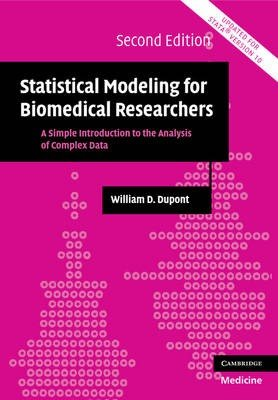Nuova legge sul prezzo dei libri - Dal 25 marzo sconti non superiori al 5%

# Statistical Modeling for Biomedical Researcherstitolo Statistical Modeling for Biomedical Researchers sottotitolo A Simple Introduction to the Analysis of Complex Data autore William D. Dupont argomento Medicina Epidemiologia e statistica medica Statistica editore Cambridge University Press formatoLibro pagine 540 pubblicazione 2009 ISBN 9780521614801
The second edition of this standard text guides biomedical researchers in the selection and use of advanced statistical methods and the presentation of results to clinical colleagues. It assumes no knowledge of mathematics beyond high school level and is accessible to anyone with an introductory background in statistics. The Stata statistical software package is again used to perform the analyses, this time employing the much improved version 10 with its intuitive point and click as well as character-based commands. Topics covered include linear, logistic and Poisson regression, survival analysis, fixed-effects analysis of variance, and repeated-measure analysis of variance. Restricted cubic splines are used to model non-linear relationships. Each method is introduced in its simplest form and then extended to cover more complex situations. An appendix will help the reader select the most appropriate statistical methods for their data. The text makes extensive use of real data sets available at http://biostat.mc.vanderbilt.edu/dupontwd/wddtext/.

### Indice testuale

Preface; 1. Introduction; 2. Simple linear regression; 3. Multiple linear regression; 4. Simple logistic regression; 5. Multiple logistic regression; 6. Introduction to survival analysis; 7. Hazard regression analysis; 8. Introduction to Poisson regression; 9. Multiple Poisson regression; 10. Fixed effects analysis of variance; 11. Repeated-measures analysis of variance; Appendix A. Summary of statistical models discussed in this text; Appendix B. Summary of Stata commands used in this text; References.